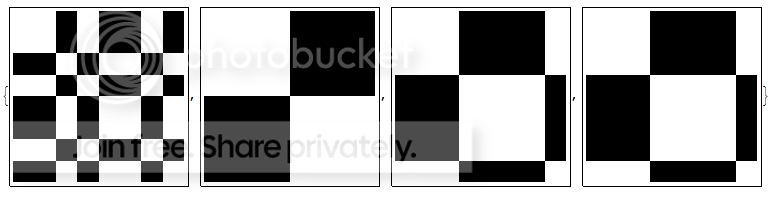# DirectAgglomerate not working for Large Matrices

I am trying to graphically display a distance matrix with similar elements visually clustered into groups.

My original matrix has the elements in a random order so the first thing I do is to change the ordering of those elements using DirectAgglomerate

Needs["HierarchicalClustering"]

Module[{indices, cluster},
indices = Range[Length @ distanceMatrix];
Reverse @ ClusterFlatten[cluster]
]


My overall function to permute the matrix then looks like this:

PermuteMatrix[distanceMatrix_, linkage_] :=
Module[{newOrder},
distanceMatrix[[newOrder, newOrder]]
]


Given an example matrix:

sampleMatrix =
{{1, 1, 0, 0, 1}, {1, 1, 0, 0, 1}, {0, 0, 1, 1, 0}, {0, 0, 1, 1, 0}, {1, 1, 0, 0, 1}};
PermuteMatrix[sampleMatrix, "Single"]

{{1, 1, 0, 0, 0}, {1, 1, 0, 0, 0}, {0, 0, 1, 1, 1}, {0, 0, 1, 1, 1}, {0 ,0, 1, 1, 1}}


The matrix is now rearranged with the similar clusters being together.

I then plot the result as a heat map while applying the same permutation to the element labels.

Everything works fine until I get to matrices over 250 x 250 in size. In this case, the Mathematica kernel crashes for some reason when evaluating DirectAgglomerate. Is this the limitation of the function or am I doing something clearly wrong?

Any help would be greatly appreciated. I am definitely no expert on clustering; perhaps there is a completely different way to go about this?

UPDATE (09.10.13)

Tried to use MinimumBandwidthOrdering as suggested by bill s but quickly ran into issues with larger matrices where groups of similar samples were broken up. Here's an example:

mSubset={{0, 0, 0.272352, 0, 0.272364, 0.272261, 0, 0.272269}, {0, 0,
0.272501, 0, 0.272514, 0.272411, 0, 0.272419}, {0.272352, 0.272501,
0, 0.272038, 0, 0, 0.272158, 0}, {0, 0, 0.272038, 0, 0.272049,
0.271947, 0, 0.271955}, {0.272364, 0.272514, 0, 0.272049, 0, 0,
0.272169, 0}, {0.272261, 0.272411, 0, 0.271947, 0, 0, 0.272068,
0}, {0, 0, 0.272158, 0, 0.272169, 0.272068, 0, 0.272075}, {0.272269,
0.272419, 0, 0.271954, 0, 0, 0.272075, 0}}

permutedMatrixOrig = PermuteMatrix[msubset, "Single"]
{r, c} = MinimumBandwidthOrdering[msubset, Method -> "Sloan"];
{r2, c2} = MinimumBandwidthOrdering[msubset, Method -> "RCMD",
RefinementMethod -> None];
{ArrayPlot[msubset],
ArrayPlot[permutedMatrixOrig],
ArrayPlot[msubset[[r, c]]],
ArrayPlot[msubset[[r2, c2]]]}I have tried playing around with the method and refinement options but was still unable to fully group all my samples. I have also noticed that for very large matrices my indices for rows and columns were different - could this have something to do with my matrices not being fully symmetrical?

• Where is ClusterFlatten defined? – bill s Sep 9 '13 at 23:42
• @bills. ClusterFlatten and DirectAgglomerate  come from the HierarchicalClustering package. I have added the omitted Needs. – m_goldberg Sep 10 '13 at 0:59

It looks like what you are trying to do is to make the matrix as "diagonal as possible" by permuting the rows and columns. There is a built-in function MinimumBandwidthOrdering that minimizes the "bandwidth" of the matrix by permuting the rows and columns, where the bandwidth is a measure of how close elements are to the diagonal. Using the same sampleMatrix defined above gives substantially the same rearrangement as in the sample.

Needs["GraphUtilities"];
{r, c} = MinimumBandwidthOrdering[sampleMatrix];
{ArrayPlot[sampleMatrix], ArrayPlot[sampleMatrix[[r, c]]]}I have used MinimumBandwidthOrdering on larger problems (1000 or so rows/columns) and never had any trouble with it. There are also has several options for the method which give related but somewhat different answers.

• Thank you for the quick reply; unfortunately the method did not work for me when I tried to apply it to larger matrices. I have updated my question with the attempt. – Msafrano Sep 10 '13 at 14:25
• @Msafrano -- I can see from your new example that what you want is not to make everything as diagonal as possible, but to separate the vertices of the graph. I might suggest either Mincut or Weakcomponents for this, but I haven't used them myself. – bill s Sep 10 '13 at 18:19